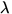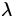# de Broglie Waves

In 1924 a young physicist, de Broglie, speculated that nature did not single out light as being the only matter which exhibits a wave-particle duality. He proposed that ordinary particles'' such as electrons, protons, or bowling balls could also exhibit wave characteristics in certain circumstances. Quantitatively, he associated a wavelengthto a particle of mass m moving at speed v :=. (10)

Since the momentum of such a particle is p = mv , mathematically this relation is equivalent to Eq.(27.8) for the momentum of a photon in Compton scattering. However, we should emphasize that these two equations have a very different physical content.

Tests of this hypothesis would involve demonstrating wave properties of matter at the wavelength given by Eq.(27.10). Relatively straightforward tests are offered by diffraction and interference - if a beam of such particles'' were shone at a diffraction grating and a diffraction pattern of a series of light and dark fringes results, then one would be forced to adopt the wave picture for this phenomena. We recall that for a good diffraction pattern to result the size of the diffraction slits should be of the same order as the wavelength of the light used. As we shall see in some examples later, for macroscopic objects such as bowling balls this would require sizes of slits of the order of 10- 34 m or so, which is much outside present-day technology. However, for electrons the sizes of slits required are of the order of 10- 11 m or so, which are readily available. Thus, it is possible to verify the wave nature of electrons in such diffraction experiments, and indeed this property is the principle behind the relatively common electron microscope. Therefore, Nature seems to be symmetric, in that light and ordinary particles'' exhibits this wave-particle duality.Next: The Uncertainty Principle Up: Quantum Physics Previous: Wave-Particle Duality